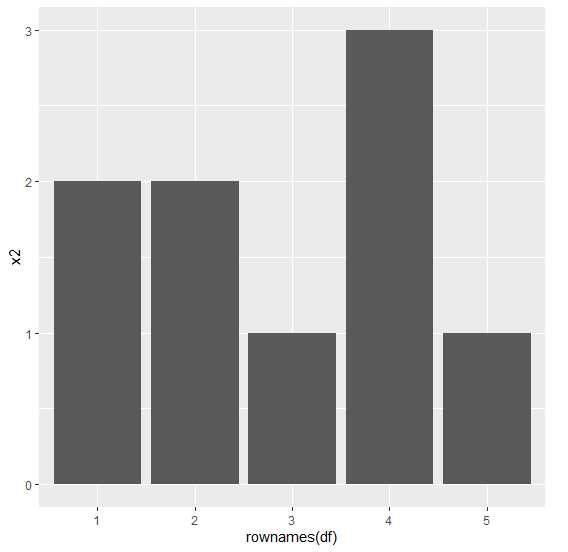# Create bar plot of one column in an R data frame using ggplot2.

To create bar plot of one column in an R data frame using ggplot2, we can use rownames of the data frame as x variable in aes.

For Example, if we have a data frame called df that contains two columns say X and Y and we want to create the bar plot of values in Y then we can use the below mentioned command −

ggplot(df,aes(rownames(df),Y))+geom_bar(stat="identity")

## Example

Following snippet creates a sample data frame −

x1<-rpois(5,10)
x2<-rpois(5,2)
x3<-rpois(5,5)
df<-data.frame(x1,x2,x3)
df

The following dataframe is created

x1 x2 x3
1 9 2 7
2 9 2 3
3 13 1 3
4 13 3 8
5 11 1 1

To load ggplot2 package and create bar plot for data in column x2 of df on the above created data frame, add the following code to the above snippet −

x1<-rpois(5,10)
x2<-rpois(5,2)
x3<-rpois(5,5)
df<-data.frame(x1,x2,x3)
library(ggplot2)
ggplot(df,aes(rownames(df),x2))+geom_bar(stat="identity")


## Output

If you execute the above given snippet, it generates the following Output −